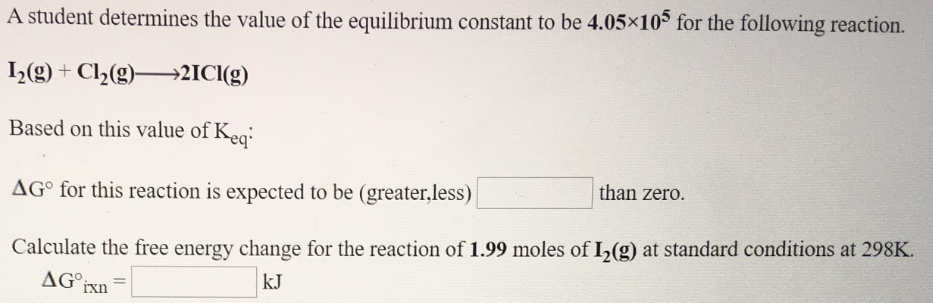# A student determines the value of the equilibrium constant to be 4.05x10^5 for the following reaction. I2(g) + Cl2(g) → 2ICl(g) Based on this value of Keq: ΔG° for this reaction is expected to be (greater, less)______ than zero. Calculate the free energy change for the reaction of 1.99 moles of I2(g) at standard conditions at 298 K.